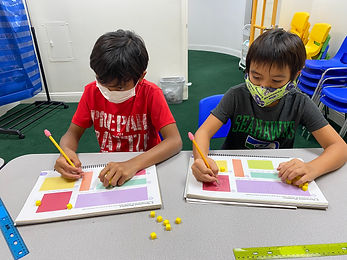## Ms. Amanda

### Target 1​

###### Lesson Type:

Continuation

Number Operation

:

Computation

Solve multi-step problems within 100 (addition, subtraction, multiplication, and division).

###### 1:

Understand that the presence of parenthesis in an arithmetic equation signals for this part of the problem to be solved first.

###### 2:

Solve problems that have more than one operation.

###### 3:

Understand when solving a problem that has both addition and subtraction operations that solving starts on the left and continues to the right.

6th

###### Vocabulary:

PEMDAS, order of operations, exponents

Activities:

Students practiced solving multi-step equations involving more than one operation and exponents.### Home Exploration

###### Guiding Questions:## Absent Students:

### Target 2

:

###### 1:

Define perimeter.

###### 2:

Understand that perimeter is the distance around a figure.

###### 3:

Understand that perimeter can be found by adding the lengths of the sides of a figure.

3rd

###### Vocabulary:

Perimeter, Sides

Activities:

- Students used centimeter cubes to find the perimeter of shapes.

- Students found the length of the sides of.a shape and added to them together to find the perimeter of the shape.

- Students were given guidelines to create a blueprint for a zoo to practice finding the perimeter and area of shapes.### Home Exploration

###### Guiding Questions:### Target 3

:

###### 1:

Recognize area as a measurement of 2D shapes.

###### 2:

Relate area to arithmetic operations (multiplication and addition).

3rd

###### Vocabulary:

Area, Length, Width

Activities:

- Students used centimeter cubes to find the area of shapes.

- Students multiplied the length of a shape by the width of the shape to find the area.

- Students were given guidelines to create a blueprint for a zoo to practice finding the perimeter and area of shapes.### Home Exploration

Find the area and perimeter of a square or rectangular shaped object at home!